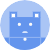search
Search
Guest 0reps
Thanks for the thanks!
close
account_circle
Profile
exit_to_app
Sign out
search
keyboard_voice
close
Searching Tips
Search for a recipe:
"Creating a table in MySQL"
Search for an API documentation: "@append"
Search for code: "!dataframe"
Apply a tag filter: "#python"
Useful Shortcuts
/ to open search panel
Esc to close search panel
to navigate between search results
d to clear all current filters
Enter to expand content previewDoc SearchCode Search BetaSORRY NOTHING FOUND!
mic
Start speaking...Voice search is only supported in Safari and Chrome.
Shrink
Navigate to
A
A
brightness_medium
share
arrow_backShareTwitterFacebook
0
thumb_down
0
chat_bubble_outline
0
auto_stories new
settings

# Pandas DataFrame | drop method

Programming
chevron_right
Python
chevron_right
Pandas
chevron_right
Documentation
chevron_right
DataFrame
chevron_right
Data Selection and Renaming
schedule Jul 1, 2022
Last updated
local_offer PythonPandas
Tags

Pandas's `DataFrame.drop(~)` method returns a DataFrame with the specified columns/rows removed.

# Parameters

1. `labels` | `string` or `list<string>` | `optional`

The name(s) of the index or column to remove.

2. `axis` | `int` or `string` | `optional`

Whether to remove columns or rows:

Axis

Meaning

Removes rows

`0` or `"index"`

Removes columns

`1` or `"columns"`

By default, `axis=0`.

3. `index`link | `string` or `list<string>` | `optional`

The name(s) of the index to remove. This is equivalent to specifying parameters `axis=0` and `labels`.

4. `columns`link | `string` or `list<string>` | `optional`

The name(s) of the columns you want to remove. This is equivalent to specifying parameters `axis=1` and `labels`.

5. `inplace`link | `boolean` | `optional`

• If `True`, then the method will directly modify the source DataFrame instead of creating a new DataFrame.

• If `False`, then a new DataFrame will be created and returned.

By default, `inplace=False`.

NOTE

For clarity, specify the parameters `index` or `columns` instead of specifying `labels` and `axis` - the code is shorter and clearer.

# Return Value

A `DataFrame` with the specified columns/rows removed. If `inplace=True`, then the return type is `None` since the source DataFrame is directly modified.

# Examples

## Dropping columns

Consider the following DataFrame:

``` df = pd.DataFrame({"A":[1,2], "B":[3,4], "C":[5,6]})df    A  B  C0  1  3  51  2  4  6 ```

### Dropping a single column

To delete column `A` only:

``` df.drop(columns="A")    B  C0  3  51  4  6 ```

This is equivalent to the following code:

``` df.drop(labels="A", axis=1)   # This is not the preferred way since the intention is unclear. ```

Note that since `inplace=False`, the original `df` is left intact.

### Dropping multiple columns

To delete columns `A` and `B`:

``` df.drop(columns=["A","B"])    C0  51  6 ```

This is equivalent to the following code:

``` df.drop(labels=["A","B"], axis=1)    # This is not the preferred way since the intention is unclear.    C0  51  6 ```

## Dropping rows

Consider the following DataFrame:

``` df = pd.DataFrame({"A":[1,2,3], "B":[4,5,6], "C":[7,8,9]})df    A  B  C0  1  4  71  2  5  82  3  6  9 ```

### Dropping a single row

To drop the second row (i.e. row with `index=1`):

``` df.drop(index=1)    A  B  C0  1  4  72  3  6  9 ```

This is equivalent to the following code:

``` df.drop(labels=, axis=0)    # This is not the preferred way.    A  B  C0  1  4  72  3  6  9 ```

### Dropping multiple rows

To drop the first two rows (i.e. rows with `index=0` and `index=1`):

``` df.drop(index=[0, 1])    A  B  C2  3  6  9 ```

This is equivalent to the following code:

``` df.drop(labels=[0,1], axis=0)    # This is not the preferred way.    A  B  C2  3  6  9 ```

## Dropping in-place

To drop row(s) or column(s) in-place, we need to set `inplace=True`. What this does is that the `drop(~)` method will directly modify the source DataFrame instead of creating and returning a new DataFrame.

As an example, consider the following DataFrame:

``` df = pd.DataFrame({"A":[1,2], "B":[3,4], "C":[5,6]})df    A  B  C0  1  3  51  2  4  6 ```

We then drop columns `A` and `B` with `inplace=True`:

``` df.drop(columns=["A", "B"], inplace=True)df    C0  51  6 ```

As shown in the output, the source DataFrame has been modified.

mail